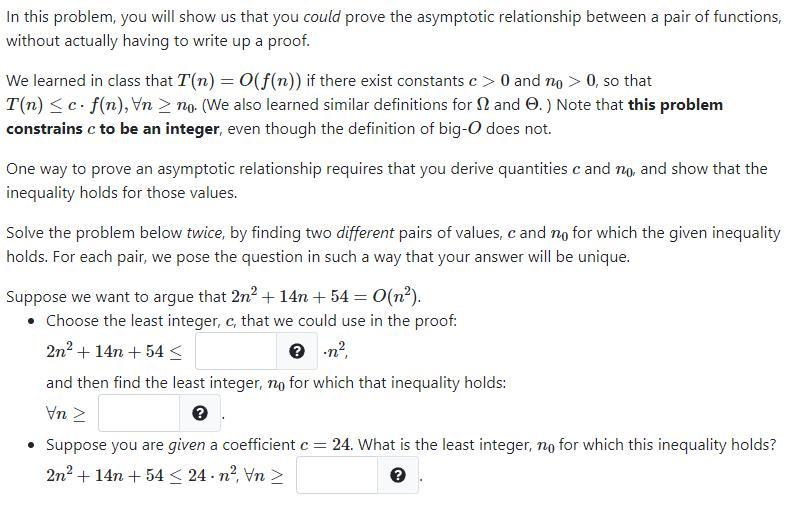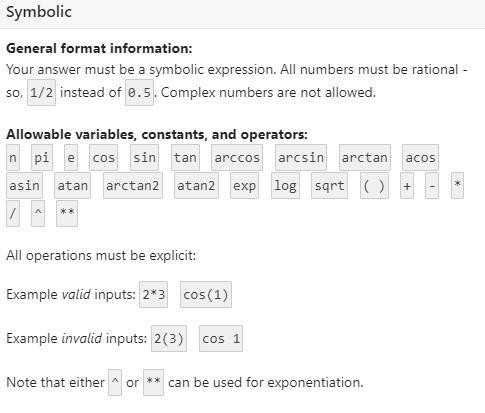# Question Please type your answer. In this problem, you will show us that you could prove the asymptotic relationship between a pair of functions, without actually having to write up a proof. We learned in class that T(n) = O(f(n)) if there exist constants c> 0 and no > 0, so that T(n) <c. f(n), Vn > no. (We also learned similar definitions for S2 and O.) Note that this problem constrains c to be an integer, even though the definition of big-O does not. One way to prove an asymptotic relationship requires that you derive quantities c and no, and show that the inequality holds for those values. Solve the problem below twice, by finding two different pairs of values, c and no for which the given inequality holds. For each pair, we pose the question in such a way that your answer will be unique. Suppose we want to argue that 2n² +14n + 54 = O(n?). • Choose the least integer, c, that we could use in the proof: 2n2 + 14n+ 54 and then find the least integer, no for which that inequality holds: Vn • Suppose you are given a coefficient c = 24. What is the least integer, no for which this inequality holds? 2n2+14n+54 < 24 - nº, Vn > Symbolic General format information: Your answer must be a symbolic expression. All numbers must be rational - so, 1/2 instead of 0.5. Complex numbers are not allowed. Allowable variables, constants, and operators: n pi e cos sin tan arccos arcsin arctan acos asin atan arctan2 atan2 explog sort O + 7 A ** All operations must be explicit: Example valid inputs: 2*3 cos (1) Example invalid inputs: 2(3) COS 1 Note that either or can be used for exponentiation.